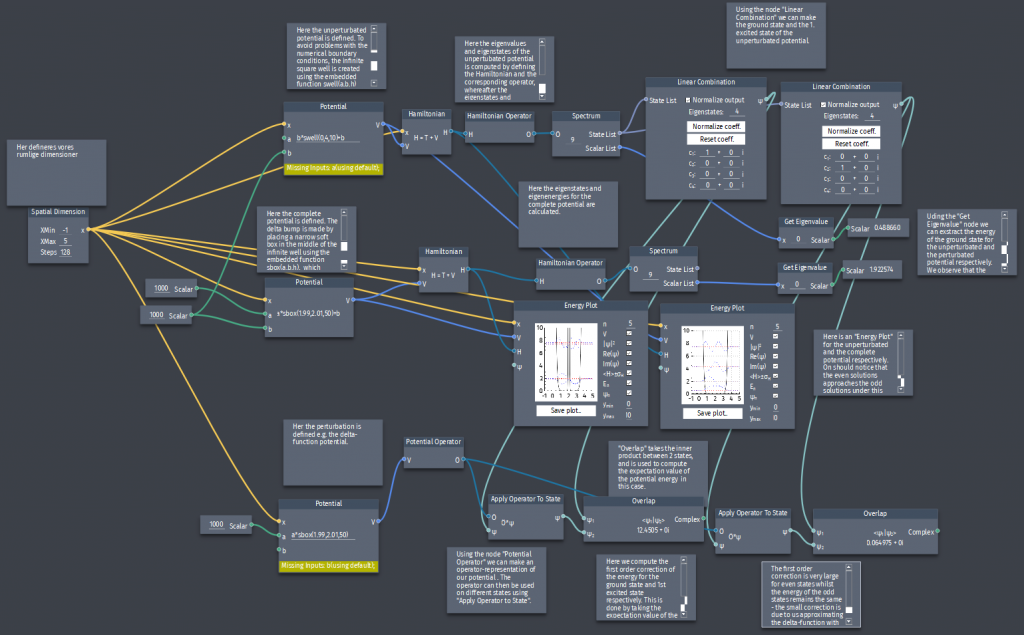# Perturbation in the infinite square well

Suppose we place a delta-function bump in the middle of the infinite square well:

$H'= \alpha \delta(x-\frac{a}{2})$

where $a$ and $\alpha$ are constants.

a) Find the 1st order corrections to the allowed energies.
b) Compare the eigenstates of the perturbated potential to those of the infinite square well.